Tip: Other languages are Google-Translated. You can visit the English version of this link.orRegisteror

## Excel SECOND function

SECOND function can help to extract second numbers from a time value. This article provides syntax and detailed sample of SECOND function to help you easily use it in Excel.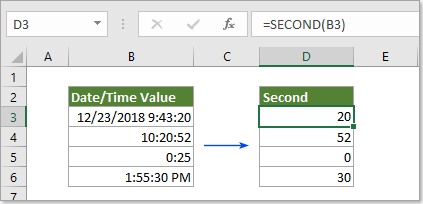Description of SECOND function

Syntax of SECOND function

Arguments of syntax

Example of SECOND function

#### Description of SECOND function

The Microsoft Excel SECOND function returns the seconds from a time value and displays as number from 0 to 59.

#### Syntax of SECOND function

=SECOND (serial_number)

#### Arguments of syntax

• Serial_number should be the valid time or date time that contains the second you will find and extract.

1) Directly reference to a cell that contains the time or date time: =SECOND(B3).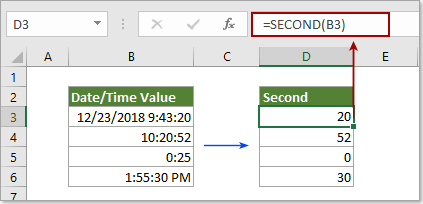2) Directly enter time as text string: =SECOND("9:43:20 AM").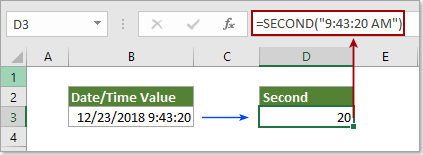3) Directly enter time as decimal numbers: =SECOND(0.405092593) (0.405092593 represents the time 9:43:20 AM).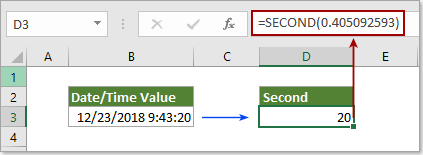4) Directly enter time as the result of a formula: =SECOND(TIMEVALUE("9:43:20 AM")).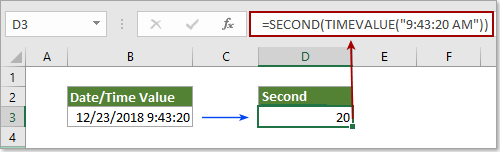#### Example of SECOND function

As below examples shown, different time formats will cause different results when using the SECOND function.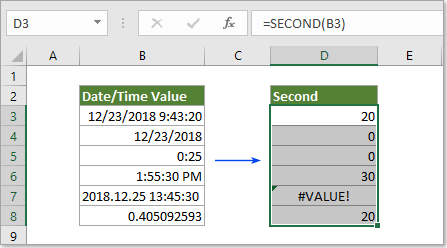Results:

 Reference Cell Data Formula Description Result 12/23/18 9:43:20 =SECOND(B3) The serial_number is a valid Time format 20 12/23/2018 =SECOND(B4) The serial_number contains no time element 0 0:25 =SECOND(B5) The serial_number contains no second element 0 1:55:30 PM =SECOND(B6) The serial_number is a valid Time format 30 2018.12.25 10:45:30 =SECOND(B7) The serial_number is an invalid time format #VALUE! 0.405092593 =SECOND(B8) The serial_number is corresponding decimal numbers 20

Remarks:

• 1. 0 occur: occurs when the serial_number contains no time or no seconds element.
• 2. #VALUE! Occur: occurs if the serial_number is an invalid Time format.Say something here...
symbols left.